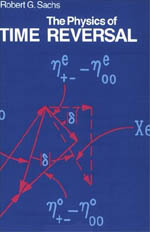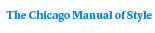# The Physics of Time Reversal

Robert G. SachsPaper \$52.00 ISBN: 9780226733319 Published October 1987
The notion that fundamental equations governing the motions of physical systems are invariant under the time reversal transformation (T) has been an important, but often subliminal, element in the development of theoretical physics. It serves as a powerful and useful tool in analyzing the structure of matter at all scales, from gases and condensed matter to subnuclear physics and the quantum theory of fields. The assumption of invariance under T was called into question, however, by the 1964 discovery that a closely related assumption, that of CP invariance (where C is charge conjugation and P is space inversion), is violated in the decay of neutral K mesons.
In The Physics of Time Reversal, Robert G. Sachs comprehensively treats the role of the transformation T, both as a tool for analyzing the structure of matter and as a field of fundamental research relating to CP violation. For this purpose he reformulates the definitions of T, P, and C so as to avoid subliminal assumptions of invariance. He summarizes the standard phenomenology of CP violation in the K-meson system and addresses the question of the mysterious origin of CP violation. Using simple examples based on the standard quark model, Sachs summarizes and illustrates how these phenomenological methods can be extended to analysis of future experiments on heavy mesons. He notes that his reformulated approach to conventional quantum field theory leads to new questions about the meaning of the transformations in the context of recent theoretical developments such as non-Abelian gauge theories, and he suggests ways in which these questions may lead to new directions of research.